Scalars and Vectors

• Last Updated : 05 Aug, 2021

In physics, quantities can be classified as scalars and vectors. These quantities differ only by direction. Vectors have a direction associated along with a magnitude with them while the scalars only have a magnitude. These are quantities that occur almost in every part of the study of the physical world. For example, the distance between two quantities can be measured and does not need a direction to express it, this is called a scalar quantity. While the movement of the particle cannot be described without using the direction in which it is moving. This is called a vector quantity. Let us study both types of quantities in detail.

Scalars and Vectors Quantities

Scalar quantities are the quantities that only have a value associated with them. These quantities can be expressed completely with a single number. Some examples of scalar quantities are – mass of an object, the distance between two points, etc. For doing operations on scalar quantities, the usual rules of algebra work. These quantities can be added and subtracted in the same way numbers are added and subtracted.

On the other hand, vectors are quantities that have a direction associated with them. For addition and subtraction, these quantities obey the triangle law of vector addition. Some examples of vector quantities include velocity, acceleration, etc. While describing the velocity, specifying the direction is necessary. Vectors are represented by, which represents the direction and the magnitude of the vector. In the case of the only magnitude, |v| denotes the magnitude. The figure below represents a vector. The length of the arrow represents the magnitude of the vector.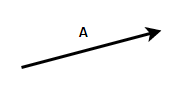Equality of Vectors

Two vectors are considered to be equal when they have the same magnitude and same direction. The figure below shows two vectors that are equal, notice that these vectors are parallel to each other and have the same length. The second part of the figure shows two unequal vectors, which even though have the same magnitude, are not equal because they have different directions.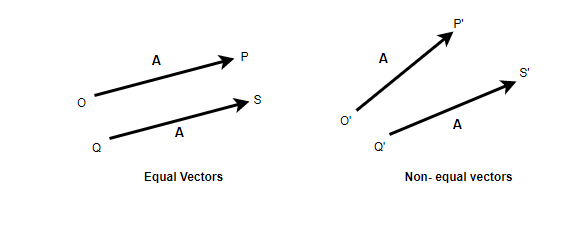Multiplication of Vectors with Scalar

Multiplying a vector a with a constant scalar k gives a vector whose direction is the same but the magnitude is changed by a factor of k. The figure shows the vector after and before it is multiplied by the constant k. In mathematical terms, this can be rewritten as,if k > 1, the magnitude of the vector increase while it decreases when the k < 1.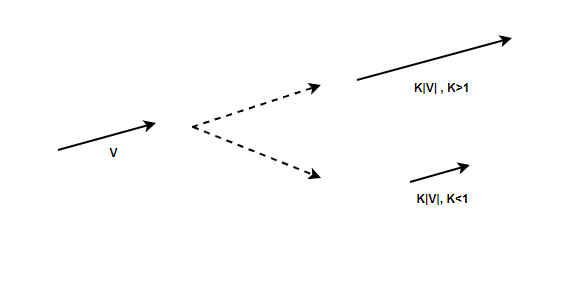Vectors cannot be added by usual algebraic rules. While adding two vectors, the magnitude and the direction of the vectors must be taken into account. Triangle law is used to add two vectors, the diagram below shows two vectors “a” and “b” and the resultant calculated after their addition. Vector addition follows commutative property, this means that the resultant vector is independent of the order in which the two vectors are added.– (Commutative Property)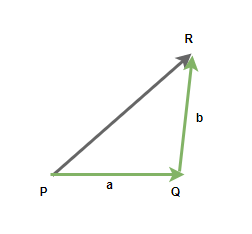Consider the vectors given in the figure above. The line PQ represents the vector “p”, and QR represents the vector “q”. The line QR represents the resultant vector. The direction of AC is from A to C.

Line AC represents,The magnitude of the resultant vector is given by,Therepresents the angle between the two vectors. Letbe the angle made by the resultant vector with the vector p.This law is just another way of understanding vector addition. This law states that if two vectors acting on the same point are represented by the sides of the parallelogram, then the resultant vector of these vectors is represented by the diagonals of the parallelograms. The figure below shows these two vectors represented on the side of the parallelogram.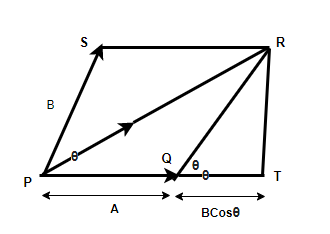Sample Problems

Question 1: Find the magnitude of v = i + 4j.

for a vector, v = ai + bj

|v| =a = 1, b = 4

|v| =⇒ |v| =⇒ |v| = √17

Question 2: A vector is given by, v = i + 4j. Find the magnitude of the vector when it is scaled by a constant of 5.

for a vector, v = ai + bj

|v| =5|v| = |5v|

a = 1, b = 4

|5v|

⇒ |5(i + 4j)|

⇒ |5i + 20j|

|v| =⇒ |v| =⇒ |v| = √425

Question 3: A vector is given by, v = i + j. Find the magnitude of the vector when it is scaled by a constant of 0.5.

for a vector, v = ai + bj

|v| =0.5|v| = |0.5v|

a = 1, b = 1

|0.5v|

⇒ |0.5(i + j)|

⇒ |0.5i + 0.5j|

|v| =⇒ |v| =⇒ |v| = √0.5

Question 4: Two vectors with magnitude 3 and 4. These vectors have a 90° angle between them. Find the magnitude of the resultant vectors.

Let the two vectors be given by p and q. Then resultant vector “r” is given by,|p| = 3, |q| = 4 and⇒⇒⇒⇒⇒ |r| = 5

Question 5: Two vectors with magnitude 10 and 9. These vectors have a 60° angle between them. Find the magnitude of the resultant vectors.

Let the two vectors be given by p and q. Then resultant vector “r” is given by,|p| = 10, |q| = 9 and⇒⇒⇒⇒Question 6: Two vectors with magnitude 4 and 4. These vectors have a 60° angle between them. Find the magnitude of the resultant vectors and the angle made by the resultant vector with 5.

Let the two vectors be given by p and q. Then resultant vector “r” is given by,|p| = 4, |q| = 4 and⇒⇒⇒⇒ |r| = 4√3⇒⇒